## Example Questions

### Example Question #21 : Geometry

Find the length of one side for a regular pentagon with a perimeter of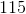.Explanation:

Use the formula for perimeter to solve for the side length: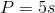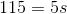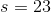### Example Question #22 : Geometry

Find the length of each side for a regular pentagon with a perimeter of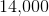.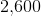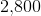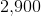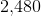Explanation:

Use the formula for perimeter to solve for the side length: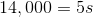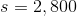### Example Question #23 : Geometry

If the side of a pentagon is, what is the diagonal of the pentagon?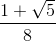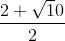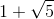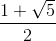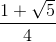Explanation:

Write the formula for finding the diagonal of a pentagon.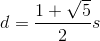Plug in the side length and simplify.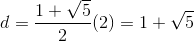### Example Question #24 : Geometry

Find the length of the diagonal for a pentagon with a a side length of.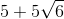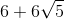Explanation:

Use the formula for the diagonal of a pentagon: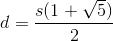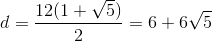### Example Question #25 : Geometry

Find the length of the diagonal for a pentagon with a perimeter of.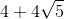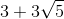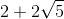Explanation:

1. Use the formula for perimeter to solve for the side length: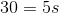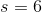2. Use the formula for the diagonal of a pentagon: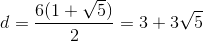### Example Question #26 : Geometry

Find the diagonal for a pentagon with a perimeter of.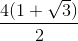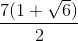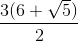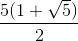Explanation:

1. Use the formula for perimeter to solve for the side length: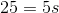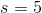2. Use the formula for the diagonal of a pentagon: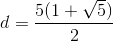### Example Question #27 : Geometry

If a side of the pentagon is, what is the diagonal of the pentagon?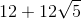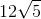Explanation:

Write the diagonal formula for a pentagon.Substitute the side length and find the diagonal.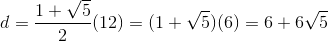### Example Question #21 : Geometry

Suppose the side length of a pentagon is. What is the area of the pentagon?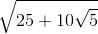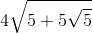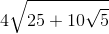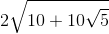Explanation:

Write the formula for the area of a pentagon.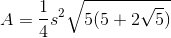Substitute the side length and simplify the radical.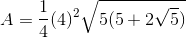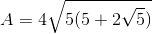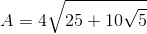### Example Question #21 : Plane Geometry

One side of a regular pentagon is. Find the perimeter of the pentagon.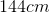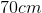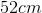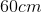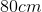Explanation:

In a regular pentagon, all of the sides are the same length.

The perimeter can then be found by summing allsides or multiplyingby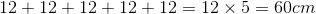### Example Question #21 : Plane Geometry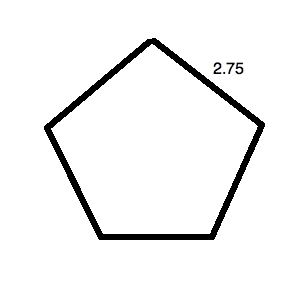What is the perimeter of the regular pentagon pictured above?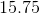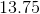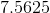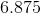Explanation:

The key word in this question is "regular." Since the pentagon is regular, all of its sides are equal. This means that it, in fact, looks like: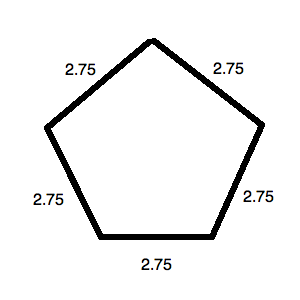The perimeter for this is simply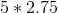or.

### All ACT Math Resources